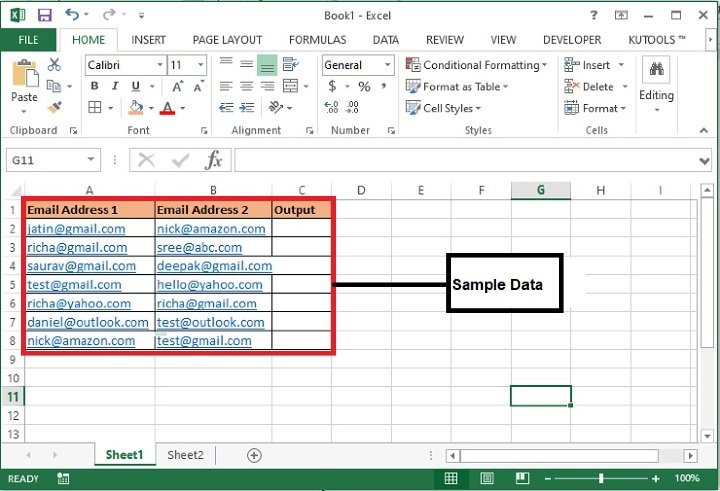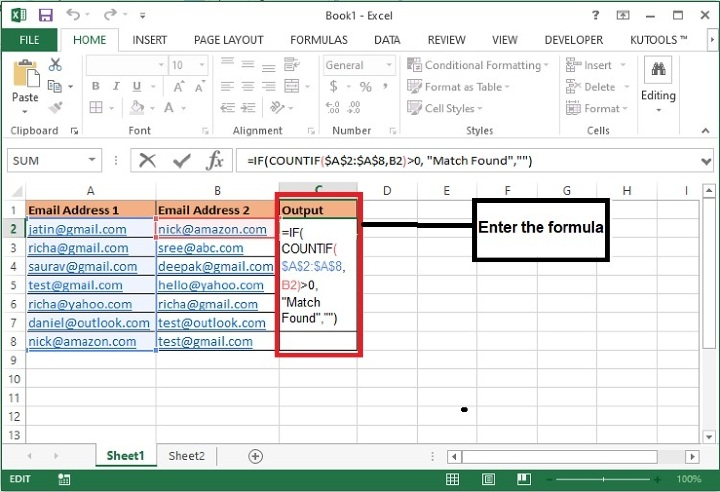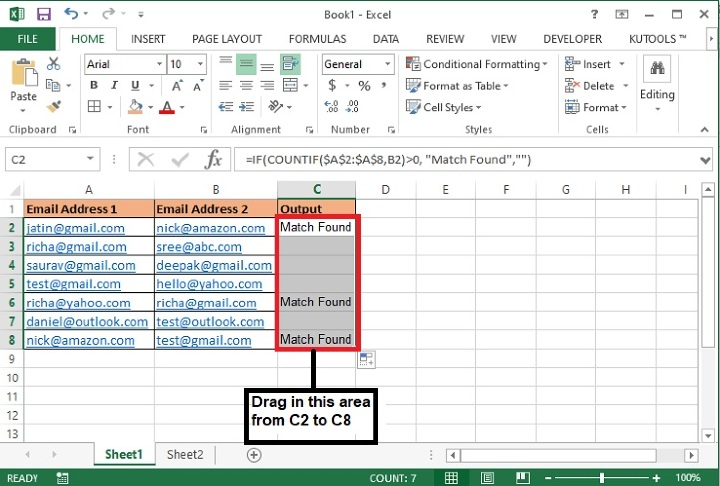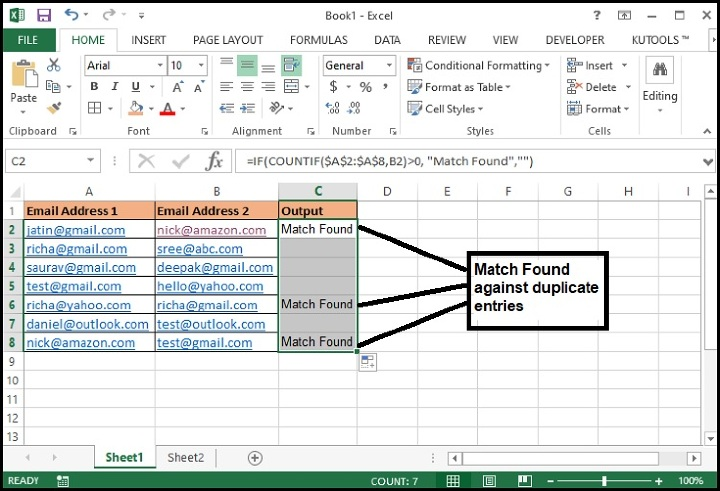# How to compare two lists of email addresses in Excel worksheet?

Sometimes to maintain the uniqueness of all values available in a workbook, we require to eliminate duplicate values from the dataset. A case of duplicity may arise when there are two columns having email addresses and you want to find the repeating ones in one of the columns. This can be achieved using the below steps.

## Comparing two columns of Email Addresses to Find the Same values using Formula

Step 1: We have taken a sample data as following. Here we have taken two columns having some email addresses and a third column in which we will display MATCH FOUND against any email address of the second row.Step 2: Now, enter this formula in the “Output” column and drag the same in other cells of the same column. This will copy the formula in all the following rows.

=IF(COUNTIF($A$2:$A$8,B2)>0, "Match Found","")


Here, the $A$2:$A$8 is the range of cells in which values of column B will be looked in. If found true, then system will print MATCH FOUND against that email address of column B.### Formula Syntax Description

Argument Description
IF(logical_test, {value_if_true},{value_if_false})

Logical_test specifies the condition basis which the data needs to be rendered.

Value_if_true specifies the value that shall be returned if the condition satisfies.

Value_if_false specifies the value that shall be returned if the condition does not satisfy.

COUNTIF(range, criteria)

Range specifies the range where you want to search the specified criteria.

Criteria specifies the data which you want to look in the defined range

Step 3: The system will display the “Match Found” against those entries of second column in which duplicate entries are available.## Conclusion

In this article we have learned how to compare two columns to identify duplicate entries of first column. This can be used to check the other values as well instead of email. Keep exploring and keep learning excel.

Updated on: 29-Aug-2023

108 Views